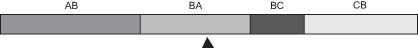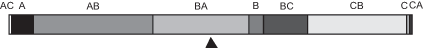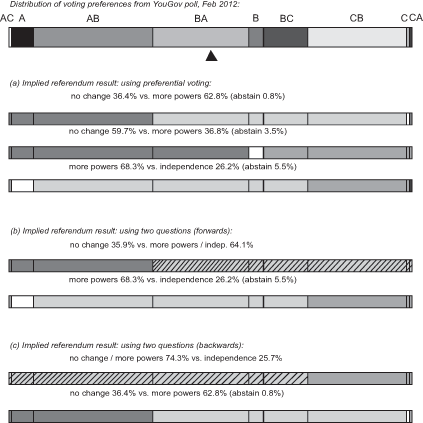# Scottish Affairs CommitteeWritten evidence submitted by Professor Denis Mollison, Heriot-Watt University

## How can the questions for a three-option referendum be put fairly and decisively?

To be the clear winner of a three-option referendum, one of the options must have a majority over each of the alternatives.

The most general way of doing this, a method that will work for any set of three options, requires either preferential voting or asking three questions.

But where the options have a natural order, as in the Scottish referendum case, a sequence of two simple Yes/No questions should be equally satisfactory. These could be asked either “forwards”, in the direction of increasing change, or “backwards”, where the first question is on independence.

A recent YouGov poll asked questions on voters’ second as well as first preferences, as between the three options of “no change”, “more powers” and “independence”. Its results confirm that the public view these options as ordered.

A crucial implication of this is that it makes barely any difference which way the referendum is structured: any of the three reasonable ways of asking the questions should give the same outcome.

Preferential: If the three options are thought of as on an equal footing, it is reasonable to demand that the winner must demonstrably command a majority when considered against each of its rivals in turn. The winner can be found through preferential voting—the voter is invited to give their first and second preferences—or by asking three questions. The latter may be thought rather long-winded, but is perhaps clearer for establishing the validity of the result.

Forwards: If we think that “devolution is a process”, then it is natural to ask two questions, the first comparing no change with change, and the second (valid only if the first yields a majority for change) comparing more powers with independence.

Backwards: If we think the most important divide is between devolution and independence, then it is natural to ask two questions, the first on independence, and the second (valid only if the first yields a majority against independence) comparing no change with more powers.

The choice between these ways of framing the questions is political, except that it would be good practice to ask an independent body such as the Electoral Commission to test whether there are significant differences from the point of view of voter understanding.

## 1. Which ways of putting the questions are fair?

We shall consider three ways of voting on the options. First, they can all be considered together, in a genuine three-option referendum; I shall refer to this structure as “preferential”, because in this case we need to consider voters’ second as well as their first preferences. Alternatively, the questions can be put in what is in effect two simple referendums in sequence—as was done in the two-question devolution referendum of 1997. There are two ways of doing this, which I shall distinguish as “forwards” and “backwards”—see below.

The three options we are considering have a natural order, from (A) no change through (B) more powers to (C) independence. So we should expect there to be few voters whose first preference is at one extreme and whose second preference is at the other. If that is true, we can think of voters as spread over a range, from the most unionist (ABs, meaning their first preference is no change and their second more powers) to the most separatist (CBs, with first preference independence and second more powers), as illustrated, with the length of each coloured bar indicating the proportion of voters with those preferences.

## THE RANGE OF EXPECTED PREFERENCESIt then follows that all three ways of asking the questions will give exactly the same result: namely, the winner is the voting preference at the midpoint of the range, where 50% want less devolution, and 50% want more (▲ in Figure 1).

So an important practical question is: do voters’ preferences follow this expected pattern? A recent YouGov poll suggests that the answer is broadly Yes.

## Preferential

If the three options are viewed as on an equal footing, then it seems reasonable to require that the winner demonstrably commands a majority when considered against each of its rivals in turn (ie is the Condorcet winner). This rules out using simple plurality (“first past the post”), the alternative vote, or the Borda count.

Perhaps the clearest way of finding the Condorcet winner is to ask three separate questions: A v. B, A v. C, and B v. C. In Peter Kellner’s graphic comparison, we can think of this as a mini soccer league. The overall winner is the option that wins both its matches.

The more usual way of finding the Condorcet winner is through preferential voting. For example, if a voter has order of preference ABC, this indicates that they prefer A to B, A to C, and B to C; if they just give a first preference A, this indicates that they prefer A to B and A to C, but abstain as to B v. C. We can thus determine the majorities for each of the three comparisons, A v. B, A v. C, and B v. C.

An obvious objection is: but what if each option wins one match? Fortunately this is extremely unlikely to happen in the present context, essentially because very few whose first preference is “no change” have second preference “independence”, or vice versa (see Appendix); but if it did happen, it seems reasonable that the winner should be deemed to be “no change”.

## Forwards

If you take the view that devolution is a process (as Donald Dewar said), in which the voters are on several occasions asked if they wish to go further, then it seems reasonable to structure the referendum as though it were two referendums telescoped into one. Thus the first question asks whether more powers are wanted, and the second, valid only if the answer to the first is Yes, asks if the voters wish to take the further step to independence.

This is the same structure as in the 1997 referendum, when the second question related to adding tax-raising powers; and is what was proposed by the SNP in their 200910 consultation. The first question in this structure is sometimes referred to as a “gateway” question.

## Backwards

If, instead, you take the view that the key division is between independence and the other two options, then it can be argued that the first (“gateway”) question should ask whether independence is wanted, and the second, valid only if the answer to the first is No, should ask if voters want more powers.

## 2. Evidence from the YouGov opinion poll

A recent YouGov opinion poll (based on questioning between 22–24 February 2012) asked a sample of 1,053 voters to give their first and second preferences as between three options:

A—Keeping the powers of Scotland’s Parliament as they are (“no change”).

B—Scotland’s Parliament having full responsibility for all taxes and spending, but within the UK (“more powers”).

C—Full independence (“independence”).

Figure 2 shows the results of this poll. As in Figure 1, the lengths of the coloured bars indicate the proportions of the sample with each combination of preferences; thus AC indicates first preference “no change” and second preference “independence”, while A indicates those with first preference “no change” and no second preference.

The great majority have preferences consistent with the ordered-options hypothesis. There are less than 1% whose first preference is at one extreme and whose second preference is at the other (AC or CA). The main difference from the expected pattern shown in figure 1 is that about 9% of those polled did not express a second preference.

## DISTRIBUTION OF VOTING PREFERENCES IN YOUGOV POLL, FEB 2012For this particular snapshot of voter opinion, the median vote (▲) is firmly in the BA region, suggesting that B (more powers) should win under any of the three ways of structuring the referendum.

This is confirmed when we consider each of those ways in detail, as is shown in Figure 3. Here the top bar simply repeats Figure 2, showing the distribution of preferences in the poll. The remaining sets of bars then show how these preferences translate into majorities for or against the various questions that might be asked.

## REFERENDUM RESULTS FOR EACH OF THE THREE WAYS OF STRUCTURING THE QUESTIONS,USING YOUGOV POLL DATA OF FEBRUARY 2012As expected, for each of the two-question formats the first question, which eliminates (at least) one of the three options, is very close to one of the preferential questions; while the second question is exactly the same as one of the preferential questions. Thus it is not surprising that all three structures lead to the same outcome, with, on these data, B winning (and the preferential format reveals that A is runner-up).

A nice irony is revealed by inspection of the detailed results. The referendum format preferred by most unionists, which keeps B off the ballot, gives the best result for independence, losing 36.8 to 59.7%. While the format (c) preferred by most nationalists gives the worst result for independence, losing 25.7 to 74.3% in the first round.

## 3. Conclusions

The YouGov poll data provides strong confirmation that all three ways of structuring a three-option constitutional referendum should yield closely similar outcomes. These three different ways correspond to different, all reasonable, ways of looking at the decision to be made; so there is no strong philosophical case for a strong preference between them.

It would therefore seem reasonable to allow the body calling the referendum, in this case the Scottish Parliament, to choose which format to adopt—with one important practical caveat. This is the question of which format is likely to be understood best by voters. An independent body, such as the Electoral Commission, could be asked to test possible question formats, and advise on whether there are significant differences in public understanding.

## CONDORCET CYCLES?

In general, there can be a problem with the preferential method, namely that there is no overall winner, because (using “A > B” to mean A has a majority against B) either C > A > B > C or A > C > B > A.

We need to explain why this cannot happen, or is at least very very unlikely, when the options have a natural order.

In A v. B, A is supported by those with AB, AC and CA preferences. In A v. C, A is supported by AB, AC and BA; we see that A has gained the support of the BAs and lost that of the CAs. But when options have a natural order, as explained in the main text, there should be a fair number of BAs but very few CAs (23.3% and 0.5% respectively in the YouGov poll). Hence if A > B, we may expect A > C by a larger margin; so we can’t have C > A > B.

Similarly, exchanging C and A in the argument, we can’t have A > C > B (this time the appropriate poll figures are BC 10.9%, AC 0.5%). This rules out both the possibilities for a Condorcet cycle.

April 2012

Prepared 4th May 2012